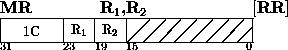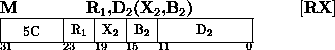Next: Multiply Halfword Up: Insert Character Previous: Move

### MultiplyThe product of the multiplier (the second operand) and the multiplicand (the first operand) replaces the multiplicand.

Both multiplier and multiplicand are 32-bit signed integers. The product is always a 64-bit signed integer and occupies an even-odd register pair. Because the multiplicand is replaced by the product, the R1 field of the instruction must refer to an even- numbered register. A specification exception occurs when R1 is odd. The multiplicand is taken from the odd register of the pair. The contents of the even numbered register replaced by the product are ignored, unless the register contains the multiplier. An overflow cannot occur.

The sign of the product is determined by the rules of algebra from the multiplier and multiplicand sign, except that a zero result is always positive.

Condition Code:
The code remains unchanged.

Program Exceptions:
Access (fetch, operand 2 of M only)
Specification

Programming Note
The significant part of the product usually occupies 62 bits or fewer. Only when two maximum negative numbers are multiplied are 63 significant product bits formed. Since two's-complement notation is used, the sign bit is extended right until the first significant product digit is encountered.Next: Multiply Halfword Up: Insert Character Previous: Move

A.Daviel,TRIUMF